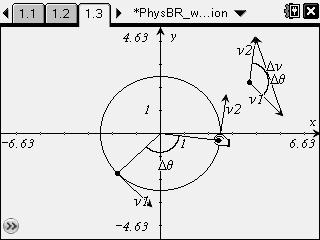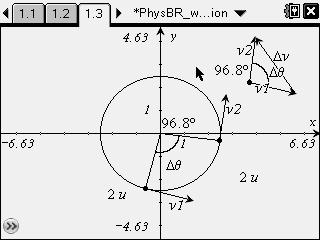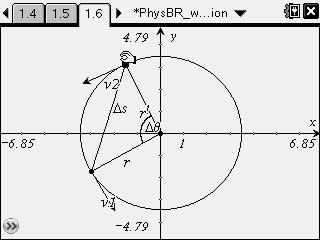# Activities

•##### Download

•• ##### Subject Area

• Science: Physics: Forces and Motion
• Science: Physics: Rotational Motion

• ##### Author9-12

15 Minutes

• ##### Device
•TI-Nspire™ CX/CX II
•TI-Nspire™ CX CAS/CX II CAS
• TI-Nspire™
• TI-Nspire™ CAS
• ##### Software

TI-Nspire™
TI-Nspire™ CAS

2.1

• ##### Report an Issue

Bell Ringer: Circular Motion

#### Activity Overview

Students observe a simulation of an object traveling in uniform circular motion.

#### Key Steps

•In this activity, students derive the equation for centripetal acceleration with respect to tangential velocity and the radius of motion. They study a diagram of a particle undergoing uniform circular motion and examine how the tangential velocity of the particle changes as it moves along its orbit.

•Students use measurement tools to confirm that the magnitude of the velocity is constant, and that the angular displacement of the particle is equal to the angle between its initial and final velocity vectors.

•Finally, students use another simulation to determine the relationship between the radius of the orbit and the straight-line displacement of the particle. They use this information, together with relationships identified in the previous situation, to derive the equation for centripetal acceleration.